# Pricing Covered Calls: A Gambler's Approach

Feb. 02, 2021 1:45 PM ET10 Comments
Please Note: Blog posts are not selected, edited or screened by Seeking Alpha editors.

Special Situations, Options, Portfolio Strategy, Growth

Contributor Since 2014

We provide options trading signals with consistent, outstanding returns.

We are nerds who love math, and we have applied our number-nerdiness to investing for years. Calculus is our co-pilot! When we discovered options selling, the light bulb went on. Since then, we have been crushing it, whether the market is good or bad.

Check us out and subscribe at www.NoGuessTrading.com.

Mom and pop operation based in Virginia.

## Summary

• Covered calls allow you to generate additional income on your long term stock positions.
• Selecting the price and strike of a covered call can be difficult.
• This article presents a quantitative way to determine whether you're being offered the right price for a covered call.

Summary

Covered calls allow you to generate additional income on your long term stock positions.

Selecting the price and strike of a covered call can be difficult.

This article presents a quantitative way to determine whether you're being offered the right price for a covered call.

Investors who leave their long stock or ETF positions sitting passively in a buy-and-hold account are missing out on opportunities to make more money. Selling covered calls is a well-known strategy for generating extra investment returns. To do so, you sell a call option on stock you already own, generally with the option strike well above the current stock price. If the option expires, you make a little money and you also get to keep your stock. This post explains what covered calls are, how they generate income, and how to find good prices for covered call trades. We have reported some real-time covered call trading on our blog.

This article describes a mathematical method for covered call pricing based on the gambling concept of "expected value," which can be implemented in Excel to determine whether the premium for a call option is enough to justify a covered call. By reviewing the options chain, the trader can rule out poorly-priced options. In this manner, the investor can complement a long stock or ETF position with the highest probability of success.

What is a call option?

A call option give you right to buy stock at a fixed price, known as the "strike price." Options are temporary; they expire. Thus, you pay a "premium" for the privilege of buying the stock at the fixed strike price sometime before expiration.

With a call option, you want the stock to rise, because you only profit when the price rises above the strike price plus the premium you paid. If the stock is higher than the strike price, then you make money because the option allows you to buy the stock at that fixed strike price while the stock is actually worth more. If the stock ends up below the strike at expiration, then your call option expires worthless. You would lose the premium that you paid for the option, but nothing more.

Example: On November 30, 2020, CVM was about \$12.50 per share, and let's say you expect it to rise. You pay \$1 per share to buy a call option for CVM at a \$35 strike that expires on January 15 -- this is denoted "CVM J15 35C." You are betting that the stock will rise above \$36 by that time. Come January 15, let's assume CVM rises to \$40 per share. You can now exercise your option and buy the stock for only \$35 per share because that is what your option lets you do. You've profited by a net \$4 per share, i.e., the \$5 difference between the \$40 price and the \$35 strike, minus the \$1 premium that you paid for the option. If CVM ends up below \$35 on January 15, then your option would expire worthless and you'd lose the \$1 per share premium. If CVM ends up between \$35 and \$36, then you lose somewhere between \$0 and \$1 per share.

Every call option pertains to 100 shares of stock. If you bought 10 call options of CVM per the example above, that would include 1000 shares at \$1 per share. Thus, for only \$1000, you can obtain the gains of 1000 shares. In the example above, if CVM closes at \$40 per share, you'd make a net of \$4 per share of \$4000 for a \$300% gain in just six weeks. Behold the power of options. Of course, if CVM didn't make it to \$35 per share, then you'd lose all \$1000, which is a 100% loss in just six weeks. Behold again the power of options. Thus, when trading options like this, you should only expose a small portion of your portfolio. Buying calls like this requires mad skills.

What's a covered call?

Covered calls, by contrast, do not require mad skills. In fact, covered calls have very low risk compared to buying calls. This is because you are selling the option, rather than buying it. Covered call writing has been my favorite trading strategy, as discussed in other posts in my blog.

A covered call is like the opposite of buying a call. With a covered call, you sell short a call option on stock you already own. Generally, the call is out of the money ("OTM"), meaning the strike is higher than the current price, like the \$35 CVM call in the example above. If the stock price doesn't get up to the option strike, then the option you sold to someone else expires worthless. And the option premium they paid goes right into your pocket. Your profits are capped at the strike price, however, if CVM continues to run up before expiration.

It is important that you own the underlying stock when selling a call, because selling calls without support from the underlying stock exposes you to unlimited downside. Example: you decide sell a CVM \$35 call for \$1. That's \$100 of premium. Yay for you. But when CVM shoots up to \$40 at expiration, the call option that you sold for just \$1 would now be worth \$40 - \$35 = \$5 per contract. At 100 shares per contact, that's \$500. If the option is exercised (it would be here), you'd be forced by your broker to buy all that stock at \$40 and sell it to the options owner for only \$35. What a mess: you lose a net of \$400, which is four times your \$100 investment. And, if CVM shot to \$60 per share, you'd be staring down a \$2400 loss.

If, however, you owned CVM stock, then you're covered. Hence the name "covered call." If CVM shot to \$60, the profit from the stock perfectly counterbalances the losses building on the call option that you sold. Whether the stock ends up at \$40 or \$60 or \$115 by January 15, your covered call would yield the same result: you earn the \$100 premium plus the stock appreciation up to \$35 and no more.

Sure, you missed out on the upside above \$35, but that's the price for writing covered calls. You trade some potential upside down the road for actual money now. And that \$100 premium isn't too shabby... at \$1 per share, it's an 8% bonus.

With a stock like CVM that might pop any minute, don't do covered calls, since you might lose out on the very reason you invested in the first place, i.e., a big gain. (Yes, I am long CVM). But with stocks or ETFs that you think might trade in a range, covered calls are definitely the way to go.

Know when to hold 'em.

Sometimes, but not always, playing poker is as easy as Kenny Rogers suggests. If you know you have a 50% chance to win a hand, and the pot of money in front of you is more than the required bet, you will place your bet every time. In the long run you will make money because the times you win outpay the times you lose.

One thing that might not be obvious is that a bet can be profitable even if you are very likely to lose. For example, say you have only a 1/6 chance to win a poker hand, and it's your turn to decide whether to call Joe Slim's \$10 bet or fold. You count the pot and you see that it's \$65. That's 6.5 times your bet. Since your chance of winning (1 out of 6) is better than what you stand to win (6.5 times your bet), you always call that bet. Five times out of six you will lose \$10 (\$50 total), but one time out of six you will win \$65. In the long run, you come out ahead. If Joe had bet \$20, then you have to fold because five times out of six you lose \$20 (\$100 total) and the one time out of six when you win only \$65 doesn't make up for those losses. As we will see, the same analysis can be applied to trading covered calls.

Casino games are usually rigged so that the house has a positive edge. Consider the roulette wheel with its 36 numbers as well as "0", and "00". There are 38 separate outcomes, but you are offered only 36 times your bet when you win. Now can see why the house always wins, a little bit at a time.

You can be the house when you sell covered calls.

Here comes the math: the concept of "expected value."

The gambling analysis above uses the concept of "expected value" (or "EV"). The EV of any bet is the sum of what you expect to win and what you expect to lose. What you expect to win is the amount of the prize times the probability of winning. What you expect to lose is the amount of the loss times the probability of losing.

In mathematical terms: EV = P(win) x \$(win) + P(loss) x \$(loss)

If the EV is positive, you will make money if you play many times. If EV is negative, then you will lose money in the long run. You won't win or lose every time, but after many plays, your average gain or loss is the EV.

An expected value example.

Say the casino has a game where you spin a big wheel with 12 colored sections on it, two of which are green. You have a 1/6th chance to win and a 5/6th chance to lose. It costs \$5 to play and you only win on green. The prize is \$20.

Do you play this game? Let's do the EV calculation.

P(win) = 1/6

\$(win) = \$15, i.e. the \$20 prize minus your \$5 bet to play.

P(loss) = 5/6

\$(loss) = -\$5.

Therefore, EV = 1/6 x \$15 + 5/6 x -\$5 = -\$1.67

You would never play this game.

The casino wants to lure more players in, at least so it can make some money from the bar, so it increases the payout to \$35. Someone at the casino will eventually be sorry:

EV = 1/6 x \$30 + 5/6 x -\$5 = \$0.83

You stand to win \$0.83 per play on average. You should play all day and all night; the casino gave you the edge.

Expanding expected value to multiple outcomes.

The above examples are based on only two possible outcomes: a win or a loss. Sometimes, there can be more than two possible outcomes, but you can still figure out the EV. Each outcome is multiplied by the probability of that outcome, and all these numbers are added up to get EV.

For instance, in the wheel game above, the casino makes another change. It offers \$15 for yellow and \$50 for green, but now it costs \$10 to play. Sounds pricey. Do you play? Again, let math be your co-pilot. Let's calculate EV by adding up all of the scenarios:

EV = 1/6 x \$40 (green) + 1/6 x \$5 (yellow) + 4/6 x -\$10 (loss) = \$0.83

Again, you still stand to win \$0.83 per play on average. You'll win some and lose some, but on average your earnings will approximate \$0.83 times the number of times you play. Stay at the table.

Investing with the EV calculation.

In the above examples, we knew the chances of winning and losing. By contrast, the stock market is not easily predicted. We don't know what the price will be in the future, and we also don't even know the probability of what the price will be in the future. We can, however, try to derive a model of the "odds" of the stock market.

And here comes the math.

Let P(\$) be a mathematical function that gives the probability that the stock will hit price \$ one month in the future. Once we have P(\$), we can then do the expected value calculation on stock prices. But what should P(\$) be?

We have found that the movement of stock prices from one month to the next generally follows what physicists call a "normal" distribution. This is the classic "bell curve" defined by its width, known as the "standard deviation" (called "S" or "σ"). A bell curve is shown below: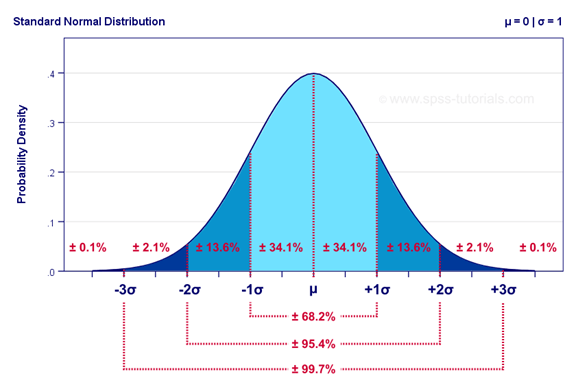Modelling the probability of stock prices with a bell curve.

The bell curve is complicated, but you can use the NORMDIST function in Excel to give you the bell curve values. For example, plug in:

= 1 - NORMDIST( [future stock price], [current stock price], [monthly standard deviation of prices], 1)

and it will give you the probability that the stock is at or above some "future stock price" in one month (assuming a flat market).

For example, on December 8, 2020, SPY closed at \$370.17 and the one-month standard deviation of price changes was 5% or about \$18.5 per share. Thus, you can use NORMDIST to estimate the probability that SPY is at least some future price in one month:

=1-NORMDIST(future price, 370.17, 18.5, 1)

You can make a table of these probabilities with Excel:

 Price in one month Probability that SPY is at least at the future price in one month 350 86.22% 354 80.90% 358 74.47% 362 67.06% 366 58.92% 370 50.37% 374 41.80% 378 33.61% 382 26.13% 386 19.61% 390 14.19% 394 9.89% 398 6.62% 402 4.27% 406 2.64% 410 1.57%

This model is flawed, however, because it ignores trends. In other words, it assumes that, on average, SPY goes nowhere. This might be true sometimes, but it's definitely not true most of the time. To improve the use of the bell curve as a model of stock prices, we can try to factor in trends. One way is to look at the average daily gain of SPY and move the center of the curve out by a month's worth of such average gains.

SPY was in an uptrend averaging 0.16% per day. One month in the future at this rate means we can expect the center of the bell curve to be approximately 0.16% x 20 = 3.2% above the current price, or 382.02.

Thus, we plug that into the NormDist as follows:

=1-NormDist(future price, 382.02, 18.5, 1)

Which yields the following predictions for SPY one month from yesterday:

 Price in one month Probability that SPY is at least at the future price in one month 350 95.83% 354 93.51% 358 90.29% 362 86.04% 366 80.67% 370 74.21% 374 66.77% 378 58.60% 382 50.04% 386 41.48% 390 33.31% 394 25.86% 398 19.39% 402 14.01% 406 9.75% 410 6.52%

Verifying the bell curve model.

This We were was skeptical at first that stock prices tended to follow the bell curve. But a quick backtest showed it to be a decent approximation. From January 1, 1994 to December 31, 2013, for SPY, the We were measured the probability distribution of prices one-month out. The We were also calculated what the NORMDIST formula above would give. The results are quite close:

 SPY one-month forward looking price distributions Standard deviations from peak Bell curve Actual SPY data Difference 0.5 38.20% 39.10% 0.90% 1 68.20% 70.70% 2.50% 1.5 86.60% 89.30% 2.70% 2 95.40% 96.20% 0.80% 2.5 98.80% 98.90% 0.10%

Using the bell curve to model your expected value.

Unlike the color-wheel game with its three scenarios, every potential future stock price has its own probability of occurring. We need to multiply the gain or loss at each price by its probability. We could do this by hand, but there is a better way: calculus. It is the Universe's amazing tool for adding up an infinity of tiny scenarios!

Written in the language of mathematics, the sum of all the gains and losses multiplied by their probabilities is an integral. Your gain or loss is the difference between the future price (called "\$") and the current price. And the probability is taken from the bell curve function P(\$). We then multiply each gain or loss by its probability and add all of that up to determine the total EV:

Equation 1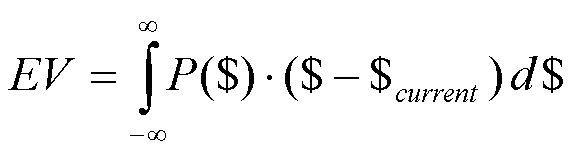Making the math useful for covered calls.

When you sell the covered call, you immediately win the premium. That's an immediate win. But, what might you you lose? Recall that profits on covered calls are capped at the strike price. Thus, any movement of the stock above the strike price is considered a loss because you give up a chance to earn those profits when you have a covered call. How much is that worth? It is simply the sum of all losses above the strike, i.e., it is equation 1 integrated (added) from the strike price up to infinity.

Thus, using our calculus friend, we can represent the total expected value with the integral equation below, with the potential win (premium) on the left and the potential loss being the fancy integral on the right:

Equation 2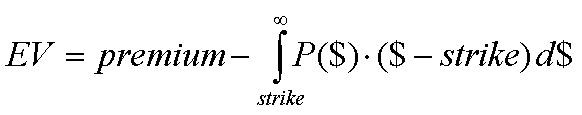As discussed above, the middle of the bell curve P(\$) is where you expect the price to be if it followed the current trend, i.e., 20 days times the average daily gain. Let's call this "U." And we also know the standard deviation of prices, which we call "S." With these values in mind, we can then show the details of P(\$), which is the probability of prices in one month:

Equation 3: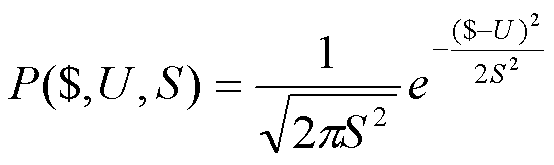I told you the bell curve was complicated. When P(\$) from Equation 3 is substituted into Equation 2, we get a final expression for the expected value based on the premium, the strike, the current price, the U, and the standard deviation S:

Equation 4: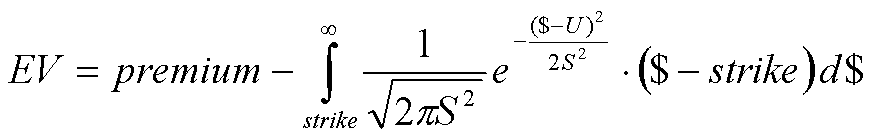That is a doozy, but it means something, and we can actually use Excel to figure it all out in the context of covered call writing.

Finding the best premium with Excel.

One wants a positive expected value when selling the covered call. Thus, we set EV > 0 in Equation 4 and, via algebraic manipulation and some of the We were's Life Force, we get an expression for what the minimum premium is:

Equation 5: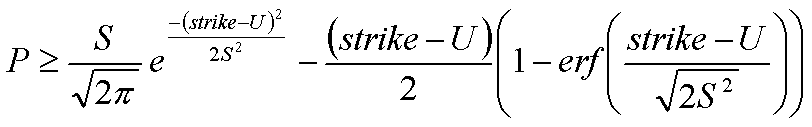The term "erf" refers to the "error function," which Excel has aptly named the "ERF" function. The P in the above equation refers to the minimum premium that the covered call must offer in order to get a positive EV from the trade.

Don't worry! It's easier than you think.

If this math looks gross, don't worry. You can actually put this into Excel as shown below. For each strike price, the formula below tells you how much premium you need in order to justify the covered call:

=S/sqrt(6.28)*exp(-1/2*power((strike-U)/S,2)) - (strike - U)/2*(1-ERF((strike-U)/sqrt(2*S*S)))

Copy and paste it into Excel and replace "U" with the cell where you have 20 x the average daily gain. Replace "S" with the cell where you have the monthly standard deviation of prices. Finally, replace "strike" with the cell where you have the strike price. If you have U in A1, S in A2, and the strike price in A3 in your Excel spreadsheet, then the formula is:

=A2/sqrt(6.28)*exp(-1/2*power((A3-A1)/A2,2)) - (A3 - A1)/2*(1-ERF((A3 A1)/sqrt(2*A2*A2)))

And now, you can start trading.

Let's do a real world application of this formula with SPY. The close on December 11, 2020 was \$366.3. The average daily gain for the last few months was 0.54 points, showing an uptrend. So we predict that the middle of the bell curve in one month will be 0.54 x 20 = 10.8 points higher than today, which is \$377.1. That's U in the equation. Then we find the monthly standard deviation of price changes (using Excel's STDEV function), which was \$18.32. This is S in the equation. Now we have all the information we need to plot the required minimum premium as a function of strike price:

The data is in the table below:

 SPY in one month Minimum premium for entering a covered call in SPY 329.00 48.14 331.00 46.15 333.00 44.16 335.00 42.18 337.00 40.20 339.00 38.23 341.00 36.28 343.00 34.33 345.00 32.40 347.00 30.49 349.00 28.60 351.00 26.74 353.00 24.91 355.00 23.12 357.00 21.37 359.00 19.66 361.00 18.01 363.00 16.42 365.00 14.89 367.00 13.44 369.00 12.05 371.00 10.75 373.00 9.53 375.00 8.40 377.00 7.35 379.00 6.39 381.00 5.51 383.00 4.72 385.00 4.02 387.00 3.39 389.00 2.84 391.00 2.36 393.00 1.94 395.00 1.59 397.00 1.28 399.00 1.03 401.00 0.82 403.00 0.65 405.00 0.50 407.00 0.39 409.00 0.30 411.00 0.23 413.00 0.17 415.00 0.13 417.00 0.09 419.00 0.07 420.00 0.06

Looking at the SPY options chain for January 15, 2021, we see the calls are much cheaper than the minimum amounts dictated by equation 5. For example, the \$35 call is only about \$15.51, but the chart says it must be at least \$23.12. The \$380 call is priced at \$1.98 but the chart says it should be more than \$5. The \$405 call is \$0.13 while the chart says it should be \$0.50. Thus, the math says that SPY is giving you a negative EV. You're likely to lose. Don't play the covered call.

Compare TRIL. If you do the same with TRIL, the story is different:

The data for TRIL is below:

 TRIL in one month Minimum premium for entering a covered call in TRIL 4.00 9.49 4.50 8.99 5.00 8.49 5.50 8.00 6.00 7.51 6.50 7.02 7.00 6.53 7.50 6.06 8.00 5.58 8.50 5.12 9.00 4.67 9.50 4.23 10.00 3.80 10.50 3.40 11.00 3.01 11.50 2.64 12.00 2.30 12.50 1.98 13.00 1.69 13.50 1.43 14.00 1.20 14.50 0.99 15.00 0.81 15.50 0.65 16.00 0.52 16.50 0.41 17.00 0.32 17.50 0.24 18.00 0.18 18.50 0.14 19.00 0.10 19.50 0.07 20.00 0.05

A January 15 TRIL call with a \$15 strike was last priced at \$1.05. This is well above the minimum premium of \$0.81 in the chart. Now you're getting positive EV, so you do the covered call on TRIL.

Limitations.

The biggest limitation of this process is assuming stocks follow the bell curve. As we saw, this was pretty good for SPY in the sample period. But it might not always hold true for SPY or for other stocks. You may choose to model stock behavior with a different probability model of future price behavior. Any probability distribution that you wish to use may be substituted as P(\$) into equation 2 and solved for the premium according to the method above.

Conclusion.

The gambling concept of expected value can be applied to the pricing of covered calls. Using equation 5, which can be implemented in an Excel spreadsheet, the minimum recommended premiums for a covered call can be estimated and used as a guide for covered call writing. Although equation 5 assumes a normal distribution of prices in the near future, the investor is not limited to a normal distribution pricing model, and can use any probability distribution model with equation 2. Good luck and happy trading!